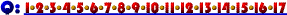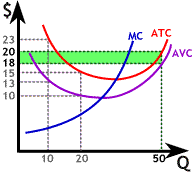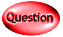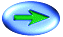10. If the firm whose costs curves are graphed to the right produces 50 units of output its Total Fixed Cost will be:

The graph to the right doesn't show fixed cost explicitly, but we can still compute Total Fixed Cost from the available information. For any given level of output we know both ATC and AVC. Since the difference between total and variable cost is just fixed cost we know that:
AFC = ATC - AVC
At 50 units of output ATC = 20 and AVC = 18, so AFC = 2. Thus
Total Fixed Cost = 50 x 2 = 100Click Here if you don't see topics listed to the left and below.